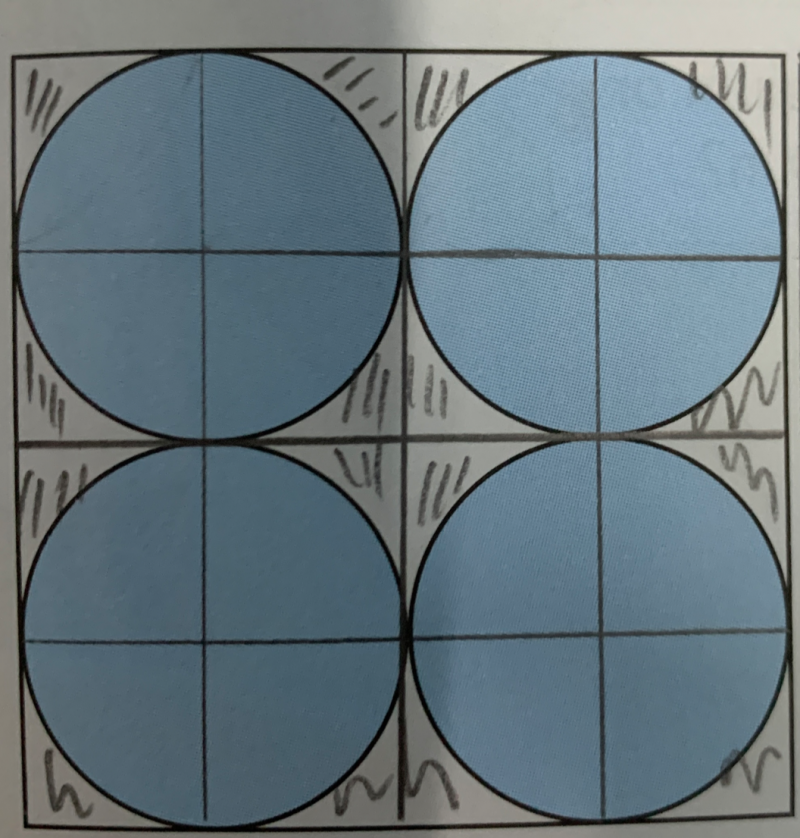# QuestionFour identical circles that touch one another lie inside a square as shown. What fraction of the square is not covered by the circles? (Take pi=22/7)

#1.  Area of Circle = πr2, Area of 4 circles = 4πr2

#2.  Area of Square:

a) Side of square = 4r

b) Area of square = (4r)2 or 16r2